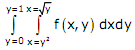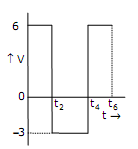# The open loop transfer function of a unity feedback system is given by G (s) = (e-01s)/s.The gain margin of this system is

1.  11.95dB

2.  17.67dB

3.  21.33dB

4.  23.9dB

4

23.9dB

Explanation :
No Explanation available for this question

# Three generators are feeding a load of 100MW.The details of the generators are Rating(MW), Efficiency(%),Regulation(p.u.)on 100 MVA base Generator-1 100 20 0.02 Generator-2 100 30 0.04 Generator-3 100 40 0.03 In the event of increased load power demand, which of the following will happen

1.  All the generators will share equal power

2.  Generator-3 will share more power compared to Generator-1

3.  Generator-1 will share more power compared to Generator-2

4.  Generator-2 will share more power compared to Generator-3

4

Generator-1 will share more power compared to Generator-2

Explanation :
No Explanation available for this question

# A 500MW,21kV,,50Hz,3-phase,2-pole synchronous generator having a rated p.f=0.9 has a moment of inertia of 27.5 x 103 kg-m2. he inertia constant (H) will be

1.  2.44s

2.  2.71s

3.  4.88s

4.  5.42s

4

2.44s

Explanation :
No Explanation available for this question

# f(x,y) is a continuous function defined over (x,y)[0,1]x[0,1].Given the two constraints,x>y2 and y>x2, the volume under f(x,y) is

1.

2.

3.

4.

4Explanation :
No Explanation available for this question

# Assume for simplicity that N people, all born in April (a month of 30 days),are collected in a room. Consider the event of at least two people in the room being born on the same date of the month,even if in different years, e.g.1980 and 1985.What is the smallest N so that the probability of this event exceeds 0.5

1.  20

2.   7

3.  15

4.  16

4

7

Explanation :
No Explanation available for this question

# A cascade of 3 Linear Time Invariant systems is causal and unstable.From this,we conclude that

1.  Each system in the cascade is individually causal and unstable

2.  At least one system is unstable and at least one system is causal

3.  At least one system is causal and all systems are unstable

4.  The majority are unstable and the majority are causal

4

At least one system is unstable and at least one system is causal

Explanation :
No Explanation available for this question

# The Fourier Series coefficient,of a periodic signal x(t),expressed as are given by a-2=2-j1;a-1=0.5+j0.2;a0=j2;a1=0.5-j 0.2;a2=2+j1;and ak=0;for |k|>2.Which of the following is true

1.  x(t) has finite energy because only finitely many coefficients are non-zero

2.  x(t) has zero average value because it is periodic

3.  The imaginary part of x(t) is constant

4.  The real part of x(t) is even

4

x(t) has finite energy because only finitely many coefficients are non-zero

Explanation :
No Explanation available for this question

# A cubic polynomial with real coefficients

1.  can possibly have no extrema and no zero crossings

2.  may have up to three extrema and up to 2 zero crossings

3.  cannot have more than two extrema and more than three zero crossings

4.  will always have an equal number of extrema and zero crossings

4

cannot have more than two extrema and more than three zero crossings

Explanation :
No Explanation available for this question

# An ideal opamp circuit and its input waveform are shown in the figures.The output waveform of this circuit will be

1.

2.

3.

4.

4Explanation :
No Explanation available for this question

# A 220V,50Hz,single-phase induction motor has the following connection diagram and winding orientations shown.MM' _ is the axis of the main stator winding (M1M2) and AA' is that of the auxiliary winding (A1A2). Directions of the winding axes indicate direction of flux when currents in the windings are in the directions shown.Parameters of each winding are indicated.When switch S is closed,the motor

1.  rotates clockwise

2.  rotates anticlockwise

3.  does not rotate

4.   rotates momentarily and comes to a halt

4